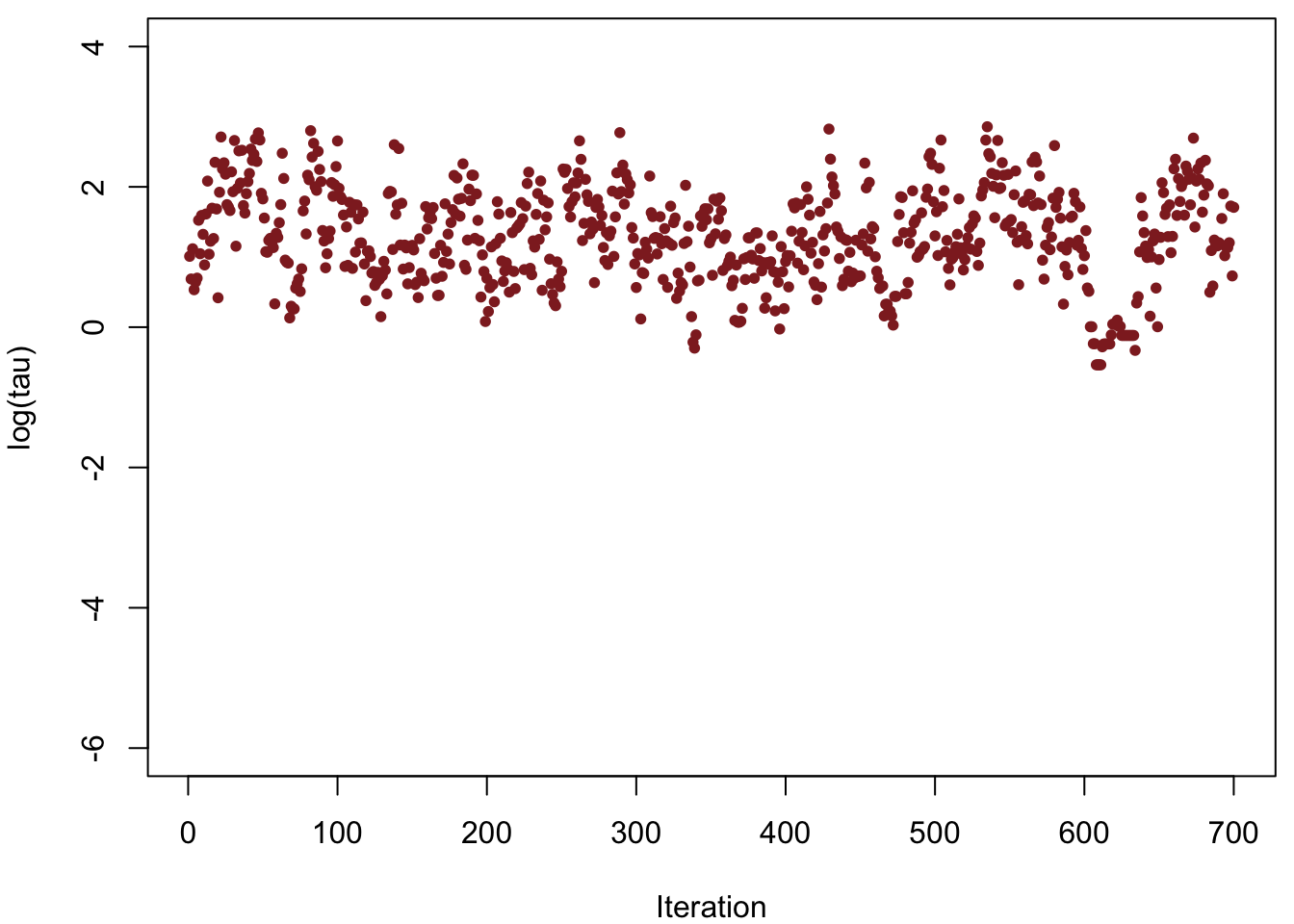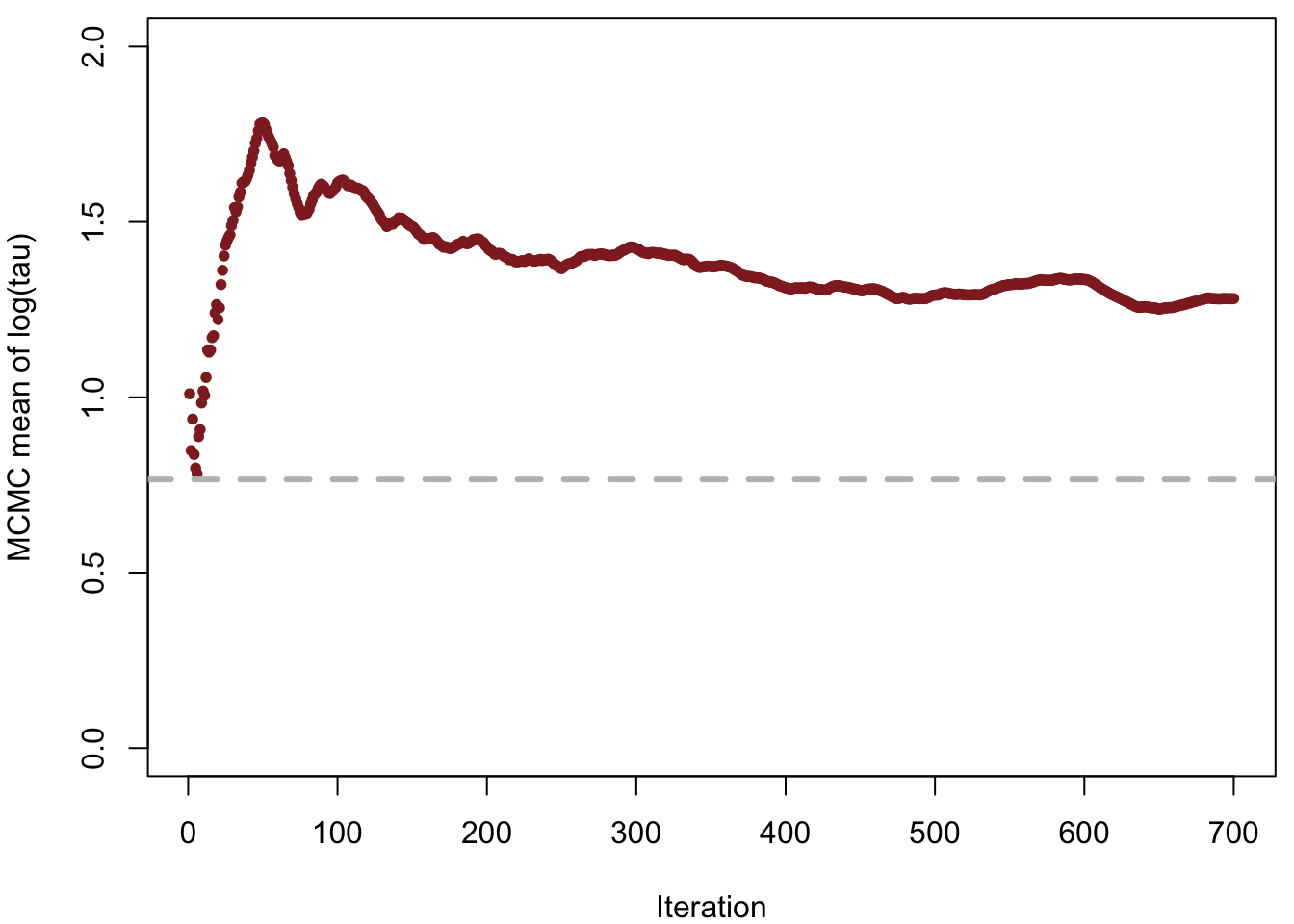Markov chain Monte Carlo (MCMC) approximates expectations with respect to a given target distribution, $\mathbb{E}_{\pi} [ f ] = \int \mathrm{d}q \, \pi (q) \, f(q),$ using the states of a Markov chain, $$\{q_{0}, \ldots, q_{N} \}$$, $\mathbb{E}_{\pi} [ f ] \approx \hat{f}_{N} = \frac{1}{N + 1} \sum_{n = 0}^{N} f(q_{n}).$ These estimators, however, are guaranteed to be accurate only asymptotically as the chain grows to be infinitely long, $\lim_{N \rightarrow \infty} \hat{f}_{N} = \mathbb{E}_{\pi} [ f ].$

To be useful in applied analyses, we need MCMC estimators to converge to the true expectation values sufficiently quickly that they are reasonably accurate before we exhaust our finite computational resources. This fast convergence requires strong ergodicity conditions to hold, in particular geometric ergodicity between a Markov transition and a target distribution. Geometric ergodicity is usually the necessary condition for MCMC estimators to follow a central limit theorem, which ensures not only that they are unbiased even after only a finite number of iterations but also that we can empirically quantify their precision using the MCMC standard error.

Unfortunately, proving geometric ergodicity theoretically is infeasible for any nontrivial problem. Instead we must rely on empirical diagnostics that identify obstructions to geometric ergodicity, and hence well-behaved MCMC estimators. For a general Markov transition and target distribution, the best known diagnostic is the split $$\hat{R}$$ statistic over an ensemble of Markov chains initialized from diffuse points in parameter space; to do any better we need to exploit the particular structure of a given transition or target distribution.

Hamiltonian Monte Carlo, for example, is especially powerful in this regard as its failures to be geometrically ergodic with respect to any target distribution manifest in distinct behaviors that have been developed into sensitive diagnostics. One of these behaviors is the appearance of divergences that indicate the Hamiltonian Markov chain has encountered regions of high curvature in the target distribution which it cannot adequately explore.

In this case study I will show how divergences signal bias in the fitting of hierarchical models and how they can be used to study the underlying pathologies. I will also show how those pathologies can be mitigated by utilizing an alternative implementation of the same model.

# The Eight Schools Model

Let’s consider a hierarchical model of the the Eight Schools dataset (Rubin 1981),

$\mu \sim \mathcal{N}(0, 5)$

$\tau \sim \text{Half-Cauchy}(0, 5)$

$\theta_{n} \sim \mathcal{N}(\mu, \tau)$

$y_{n} \sim \mathcal{N}(\theta_{n}, \sigma_{n}),$

where $$n \in \left\{1, \ldots, 8 \right\}$$ and the $$\left\{ y_{n}, \sigma_{n} \right\}$$ are given as data.

Inferring the hierarchical hyperparameters, $$\mu$$ and $$\sigma$$, together with the group-level parameters, $$\theta_{1}, \ldots, \theta_{8}$$, allows the model to pool data across the groups and reduce their posterior variance. Unfortunately this pooling also squeezes the posterior distribution into a particularly challenging geometry that obstructs geometric ergodicity and hence biases MCMC estimation.

In this case study we’ll first examine the direct centered parameterization of the Eight Schools model and see how divergences identify this bias before there are any other indications of problems. We’ll then use these divergences to study the source of the bias and motivate the necessary fix, a reimplementation of the model with a non-centered parameterization. For a more thorough discussion of the geometry of centered and non-centered parameterizations of hierarchical models see Betancourt and Girolami (2015).

# A Centered Eight Schools Implementation

A centered parameterization of the Eight Schools model is straightforward to directly implement as a Stan program,

writeLines(readLines("eight_schools_cp.stan"))
data {
int<lower=0> J;
real y[J];
real<lower=0> sigma[J];
}

parameters {
real mu;
real<lower=0> tau;
real theta[J];
}

model {
mu ~ normal(0, 5);
tau ~ cauchy(0, 5);
theta ~ normal(mu, tau);
y ~ normal(theta, sigma);
}

Unfortunately, this direct implementation of the model exhibits a pathological geometry that frustrates geometric ergodicity. Even more worrisome, the resulting bias is subtle and may not be obvious upon inspection of the Markov chain alone. To understand this bias, let’s consider first a short Markov chain, commonly used when computational expediency is a motivating factor, and only afterwards a longer Markov chain.

## A Dangerously-Short Markov Chain

We begin by setting up our R environment,

library(rstan)
Loading required package: ggplot2
Loading required package: StanHeaders
rstan (Version 2.14.1, packaged: 2016-12-28 14:55:41 UTC, GitRev: 5fa1e80eb817)
For execution on a local, multicore CPU with excess RAM we recommend calling
rstan_options(auto_write = TRUE)
options(mc.cores = parallel::detectCores())
rstan_options(auto_write = TRUE)

and then some graphic customizations that we’ll use later,

c_light <- c("#DCBCBC")
c_light_highlight <- c("#C79999")
c_mid <- c("#B97C7C")
c_mid_highlight <- c("#A25050")
c_dark <- c("#8F2727")
c_dark_highlight <- c("#7C0000")

Against the best practices preached by the Stan development team, let’s fit the model in RStan using just a single short chain,

input_data <- read_rdump("eight_schools.data.R")

fit_cp <- stan(file='eight_schools_cp.stan', data=input_data,
iter=1200, warmup=500, chains=1, seed=483892929, refresh=1200)

SAMPLING FOR MODEL 'eight_schools_cp' NOW (CHAIN 1).

Chain 1, Iteration:    1 / 1200 [  0%]  (Warmup)
Chain 1, Iteration:  501 / 1200 [ 41%]  (Sampling)
Chain 1, Iteration: 1200 / 1200 [100%]  (Sampling)
Elapsed Time: 0.054132 seconds (Warm-up)
0.048684 seconds (Sampling)
0.102816 seconds (Total)

Although this scenario may appear superficial, it is not uncommon for users to use short chains when prototyping their analysis, or even for their final analysis if they are limited by time or computational resources.

For this lone chain split $$\hat{R}$$ doesn’t indicate any problems and the effective sample size per iteration is reasonable,

print(fit_cp)
Inference for Stan model: eight_schools_cp.
1 chains, each with iter=1200; warmup=500; thin=1;
post-warmup draws per chain=700, total post-warmup draws=700.

mean se_mean   sd   2.5%    25%    50%    75% 97.5% n_eff Rhat
mu         4.29    0.26 3.29  -2.99   2.06   4.53   6.33 10.86   165 1.00
tau        4.50    0.37 3.09   0.89   2.27   3.54   5.94 13.05    70 1.01
theta   6.60    0.34 5.73  -3.59   2.95   6.21   9.52 19.25   281 1.00
theta   5.28    0.31 4.90  -4.72   2.50   5.44   7.83 15.27   249 1.01
theta   3.83    0.33 5.77 -10.62   0.91   4.29   7.16 14.36   311 1.00
theta   4.99    0.30 5.25  -5.53   1.98   4.96   8.28 15.13   302 1.00
theta   3.37    0.37 5.13  -7.39   0.22   4.00   6.36 13.44   192 1.00
theta   3.77    0.36 5.02  -7.33   0.99   4.08   6.90 12.81   200 1.00
theta   6.87    0.38 5.51  -3.17   3.28   6.09   9.60 20.43   206 1.01
theta   4.68    0.28 5.25  -6.01   1.80   4.81   7.36 15.53   341 1.00
lp__     -16.77    0.83 5.42 -26.88 -20.65 -16.91 -13.14 -5.12    43 1.02

Samples were drawn using NUTS(diag_e) at Thu Mar  2 15:24:17 2017.
For each parameter, n_eff is a crude measure of effective sample size,
and Rhat is the potential scale reduction factor on split chains (at
convergence, Rhat=1).

Moreover, the trace plots all look fine. Let’s consider, for example, the hierarchical standard deviation, $$\tau$$ or, more specifically, its logarithm, $$\log \tau$$. Because $$\tau$$ is constrained to be positive, its logarithm will allow us to better resolve behavior for small values. Indeed the chains seems to be exploring both small and large values reasonably well,

params_cp <- as.data.frame(extract(fit_cp, permuted=FALSE))
names(params_cp) <- gsub("chain:1.", "", names(params_cp), fixed = TRUE)
names(params_cp) <- gsub("[", ".", names(params_cp), fixed = TRUE)
names(params_cp) <- gsub("]", "", names(params_cp), fixed = TRUE)
params_cp$iter <- 1:700 par(mar = c(4, 4, 0.5, 0.5)) plot(params_cp$iter, log(params_cp$tau), col=c_dark, pch=16, cex=0.8, xlab="Iteration", ylab="log(tau)", ylim=c(-6, 4))Unfortunately, the resulting estimate for the mean of $$log(\tau)$$ is strongly biased away from the true value, here shown in grey, running_means <- sapply(params_cp$iter, function(n) mean(log(params_cp$tau)[1:n])) par(mar = c(4, 4, 0.5, 0.5)) plot(params_cp$iter, running_means, col=c_dark, pch=16, cex=0.8, ylim=c(0, 2),
xlab="Iteration", ylab="MCMC mean of log(tau)")
abline(h=0.7657852, col="grey", lty="dashed", lwd=3)Hamiltonian Monte Carlo, however, is not so oblivious to these issues as almost 2% of the iterations in our lone Markov chain ended with a divergence,

divergent <- get_sampler_params(fit_cp, inc_warmup=FALSE)[][,'divergent__']
sum(divergent)
 12
sum(divergent) / 700
 0.01714286

Even with a single short chain these divergences are able to identity the bias and advise skepticism of any resulting MCMC estimators.

Additionally, because the divergent transitions, here shown here in green, tend to be located near the pathologies we can use them to identify the location of the problematic neighborhoods in parameter space,

params_cp$divergent <- divergent div_params_cp <- params_cp[params_cp$divergent == 1,]
nondiv_params_cp <- params_cp[params_cp$divergent == 0,] par(mar = c(4, 4, 0.5, 0.5)) plot(nondiv_params_cp$theta.1, log(nondiv_params_cp$tau), col=c_dark, pch=16, cex=0.8, xlab="theta.1", ylab="log(tau)", xlim=c(-20, 50), ylim=c(-6,4)) points(div_params_cp$theta.1, log(div_params_cp\$tau),
col="green", pch=16, cex=0.8)